# EF2 – Digital Shapes

## Smooth Discrete Surfaces

Project Members

Project Duration

01.01.2019 – 31.12.2021

Located at

### Description

Polyhedral surfaces are fundamental objects in architectural geometry and industrial design. The closeness of a given mesh to a smooth reference surface and its suitability for numerical simulations were already studied extensively. However, suitable assessments of the smoothness of a polyhedral surface are a very recent direction of research.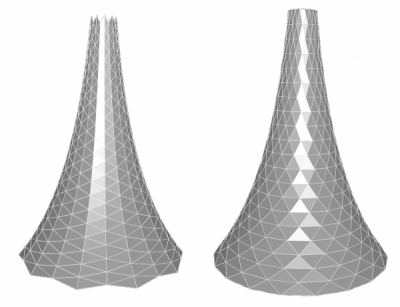The pictures above show a smooth and a non-smooth discretization of a cone-like surface where smoothness is assessed by their reflection patterns. Smooth polyhedral surfaces are not only relevant in an architectural context, where vertices and edges of polyhedral surfaces are highly visible. We also developed a rich mathematical theory of smooth discrete surfaces. This includes complimentary notions of smoothness and discrete curvatures of polyhedral surfaces, as well as a deeper understanding of discrete curvature flows. The main results of our project Smooth discrete surfaces are:

• We proved a discrete Theorema Egergium for polyhedral vertex stars. This allowed us to describe the possible Gauss images.
• We developed a theory of smooth polyhedral surfaces that is invariant under projective transformations.
• We initiated a curvature theory of general polyhedral surfaces and connected it to new definitions of smoothness of polyhedral surfaces that are invariant under affine transformations.
• We studied discrete mean curvature flows and proved the convergence of convex polygons under the area-preserving discrete curve shortening flow in the general anisotropic setting.

Further Details

In differential geometry, Gaussian curvature can be computed in several equivalent ways. One option is to consider Gaussian curvature as the local change of signed area under the Gauss map. This map associates to each point of an oriented neighborhood of the smooth surface its outer spherical normal vector. Since this map makes use of a local embedding of the surface into an ambient space, it gives
rise to an extrinsic definition of Gaussian curvature. Gauss’ famous Theorema Egregium states that the Gaussian curvature of a smooth surface is actually an intrinsic quantity. This means that it can be computed by measurements of distances that take place just on the surface.

The discrete Theorema Egregium for polyhedral surfaces can be stated as follows: The (extrinsic) algebraic area of the Gauss image of a polyhedral vertex star equals its (intrinsic) angle deficit, known as discrete Gaussian curvature. We found an elementary proof of this theorem. The heart of the proof is the comparison of the winding numbers of a spherical normal vector and its antipodal vector around the Gauss image with its critical point index.

A consequence of the theorem is that the number of inflection faces of a polyhedral vertex star is related to the numbers of positive and negative components in the Gauss image. The resulting formula significantly limits the possible shapes of Gauss images. In particular, a Gauss image of a polyhedral vertex star that has no self-intersections is either a convex spherical polygon if the curvature is positive or a spherical pseudo-quadrilateral if the curvature is negative. When we consider vertex stars with non-convex faces, just spherical pseudo-triangles and -digons may appear as well, see the figure below.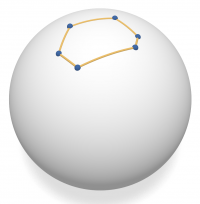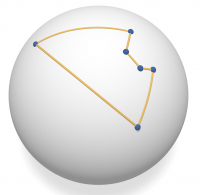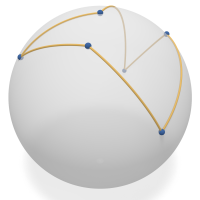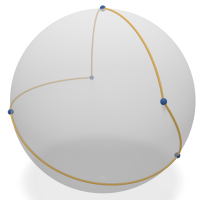We introduced notions of smoothness for polyhedral surfaces starting with the property that the Gauss image of a vertex star has no self-intersections. To get further analogies to the smooth theory, we additionally required that the Gauss image of each negatively curved vertex star is star-shaped. This condition can be even easier implemented into an algorithm making the theory accessible to applications in architecture and computer graphics. The notion of smoothness that we developed is projectively invariant and takes into account how the Gauss images of vertex stars are arranged around a face.

In this setting, projective transformations map the discrete normals, discrete tangent planes, discrete asymptotic directions, and discrete parabolic curves to the corresponding objects of the image surface. Even more, the projective dual of a smooth polyhedral surface is smooth as well and its Gauss image is the projection of the original surface to the sphere, see the figure below. This explains why both in the Gauss image and in smooth polyhedral surfaces spherical pseudo-quadrilaterals in regions of negative curvature are observed.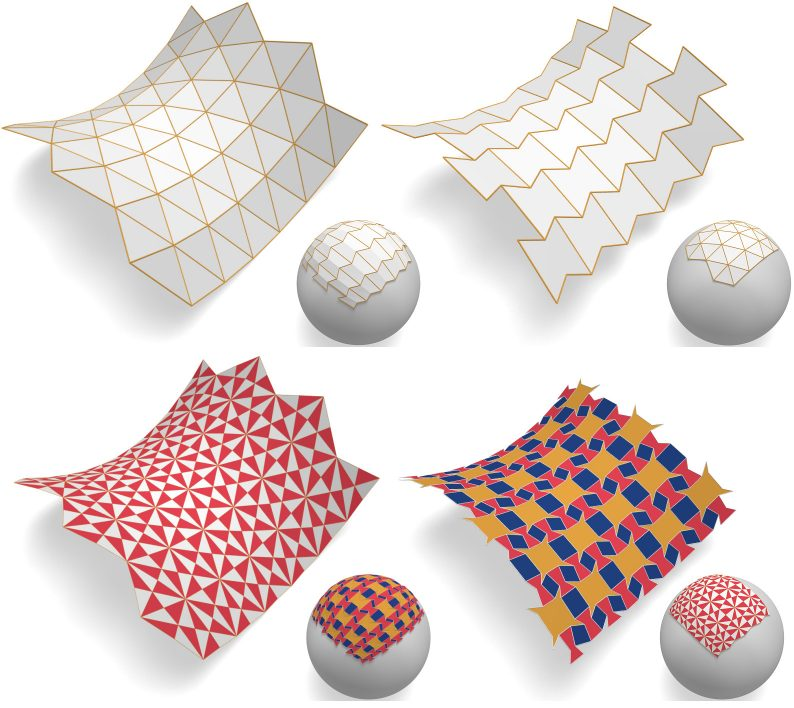We further have been developing a curvature theory for general polyhedral surfaces based on Steiner’s formula. We generalized the previously known approach to surfaces whose face offsets do not have the same combinatorics. By splitting vertices of higher valence into trees, we defined a discrete mean curvature and a discrete Gaussian curvature on a triple of an incident vertex, edge, and face. These curvatures satisfy a discrete analog of Steiner’s formula.

While the discrete mean curvature does not depend on the particular splitting of the vertices, the discrete Gaussian curvature does. Nevertheless, these discrete Gaussian curvatures are the gradients of the discrete mean curvature of the corresponding offset surfaces. In turn, the discrete mean curvature is the gradient of the surface area. These are the exact analogs to the smooth case.

Focusing on polyhedral surfaces that discretize either negatively or positively curved surfaces, we introduced the following new notions of smoothness: A weak notion of smoothness is fulfilled if the polyhedral Gauss image is free of self-intersections; a strong notion of smoothness is fulfilled if in addition, all discrete Gaussian curvatures have the same sign. The weak notion of smoothness is equivalent to the spherical Gauss image being extendable to a spherical Delaunay triangulation, whereas the strong notion of smoothness is equivalent to the property that the edges of the Voronoi diagram of the normal vectors are intersecting the edges of the spherical Gauss image in their interior. In particular, faces of the polyhedral Gauss image are star-shaped with respect to the point of contact with the unit sphere.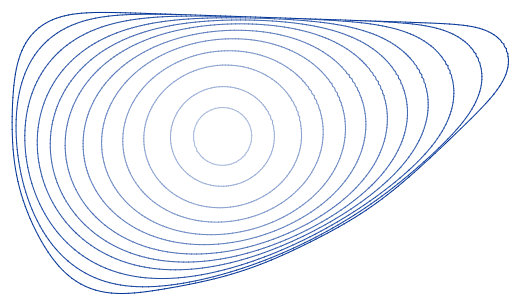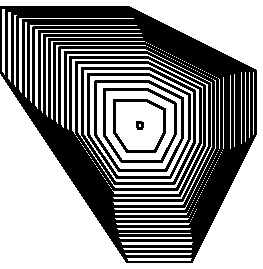Curve shortening flows and mean curvature flows were intensively studied and found applications in material sciences and computer science. It is known that under the curve shortening flow, any smooth embedded curve shrinks to a point in finite time. The limiting shape is a circle. For the curve shortening flow in Minkowski geometry however, a family of convex curves evolving under the flow shrinks to a point in finite time, but if the family of curves is renormalized to enclose constant area, just each infinite sequence of curves has a convergent subsequence which converges to the shape of a self-similar solution. The latter is unique if the original flow was symmetric with respect to the origin, but there exist two distinct homothetically shrinking solutions for a particular curve shortening flow. Another important instance of the curve shortening flow is its area-preserving version. A simple closed convex plane curve that evolves according to that flow remains so for all times and converges to a circle in infinite time. In higher dimensions, it is known that convex surfaces converge to a point in finite time under the mean curvature flow and the limiting shape is a sphere. But almost nothing is known for the anisotropic case yet. However, the (anisotropic) volume-preserving mean curvature flow behaves well and convex surfaces converge to the corresponding anisotropic unit sphere in infinite time.

We studied discretizations of these flows. We discretized the mean curvature using Steiner’s formula and investigated the corresponding flows moving edges of convex polygons or faces of convex polyhedra parallelly, see the figure above. It turns out that such flows are already known as crystalline flows. The discrete unit circles are then called Wulff shapes. We confirmed that every convex polygon shrinks to a point in finite time under the discrete curve shortening flow. In addition, we found counterexamples to the hypothesis that every convex polygon with more than four vertices shrinks to a Wulff shape shaped point. For the analysis of discrete curve shortening flows, we developed a software suitable for experiments. We want to adapt this software to the discrete mean curvature flow of polyhedra as well. For that flow, counterexamples are known, but no general class of Wulff shapes for which convergence holds is known up to now.

In contrast, we found a new and instructive proof that under the discrete area-preserving curve-shortening flow convex polygons converge to the Wulff shape. To our knowledge, this is the first proof in the general anisotropic setting. The key idea is to apply the Cauchy-Schwarz identity (rather than just the inequality) to the time derivative of the perimeter. We hope that a similar ansatz applies to the discrete volume-preserving mean curvature flow for convex polyhedra such that we can confirm the yet open hypothesis of convergence of convex polyhedra under this flow.

Selected Publications

T. Banchoff, F. Günther. The Gauss map of polyhedral vertex stars, 35 pages, 2019. Preprint arXiv:1909.09184.

F. Günther, C. Jiang, H. Pottmann. Smooth polyhedral surfaces, Adv. Math. 363, 107004, 31 pages, 2020.

Selected pictures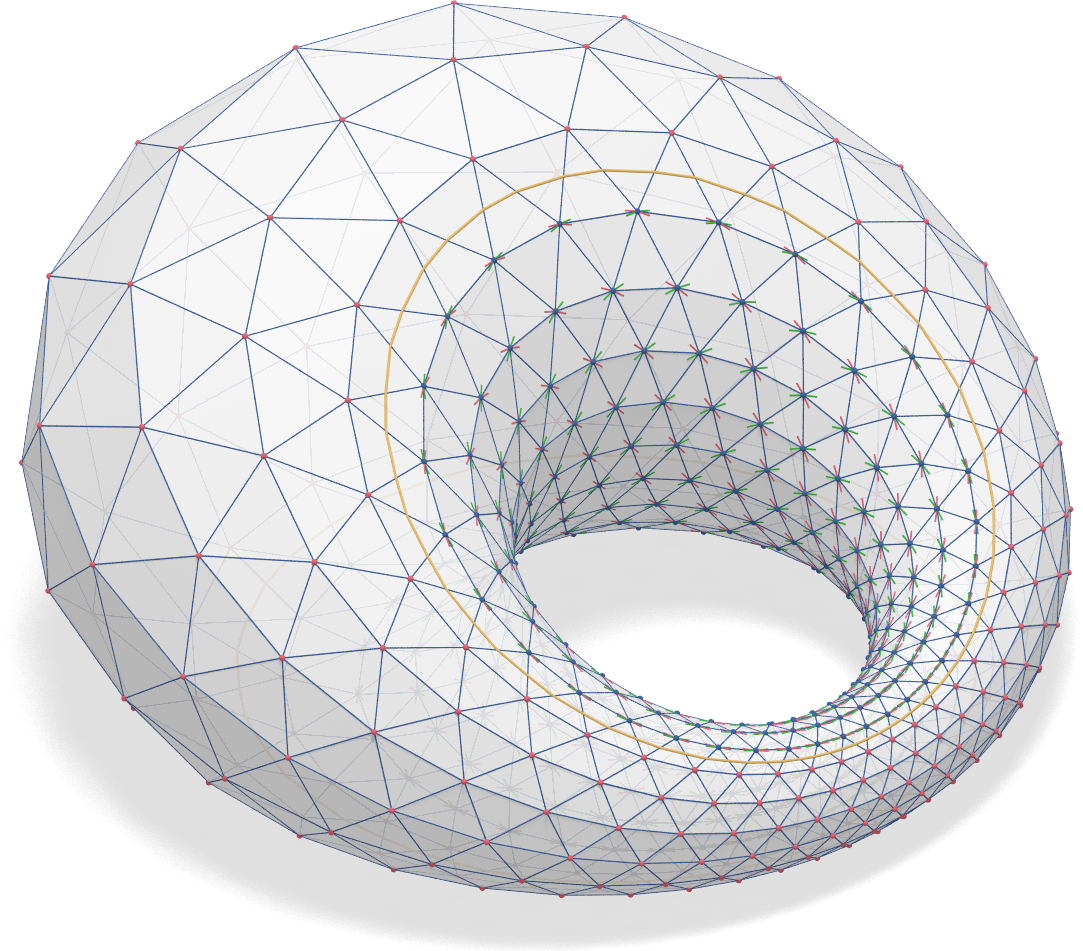A smooth discrete Dupin cyclide composed of triangles: The discrete parabolic curve is yellow, the discrete asymptotic directions are marked red and green.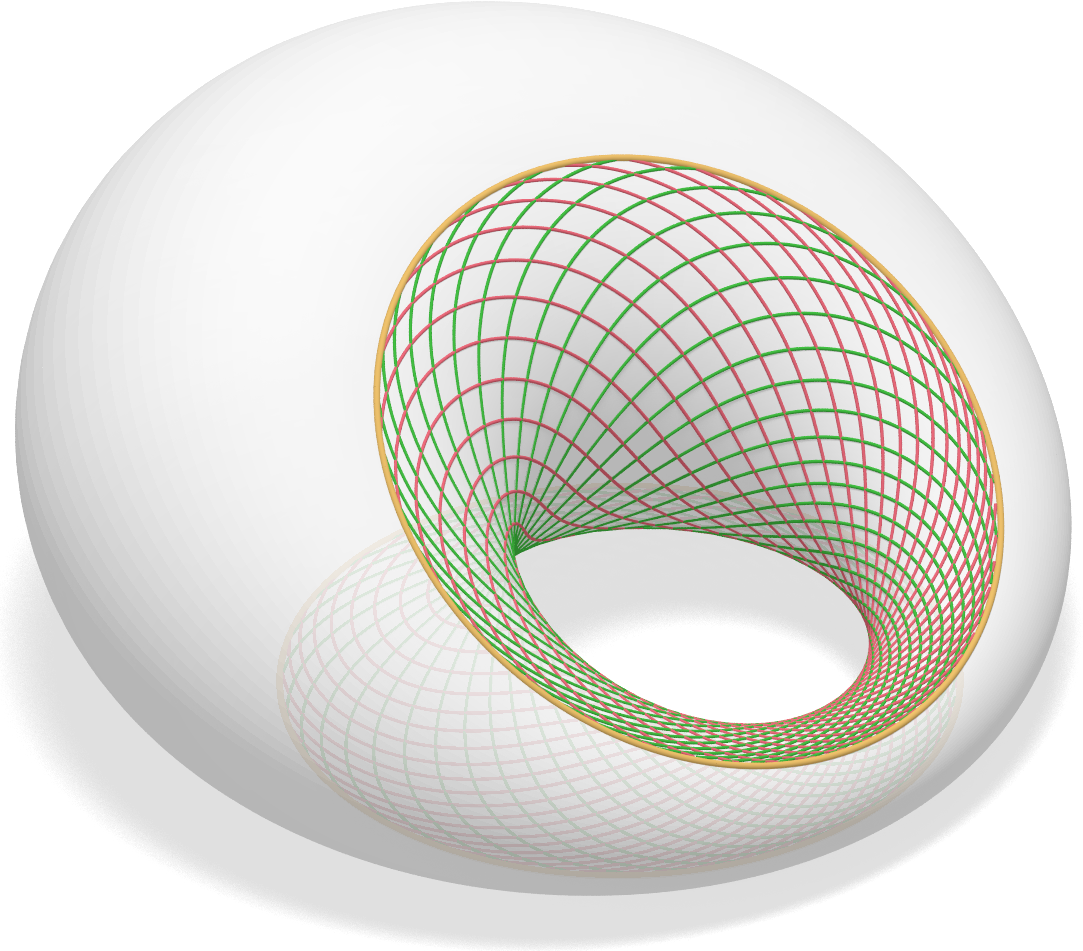A smooth Dupin cyclide: The parabolic curve is yellow, two families of asymptotic directions are marked red and green.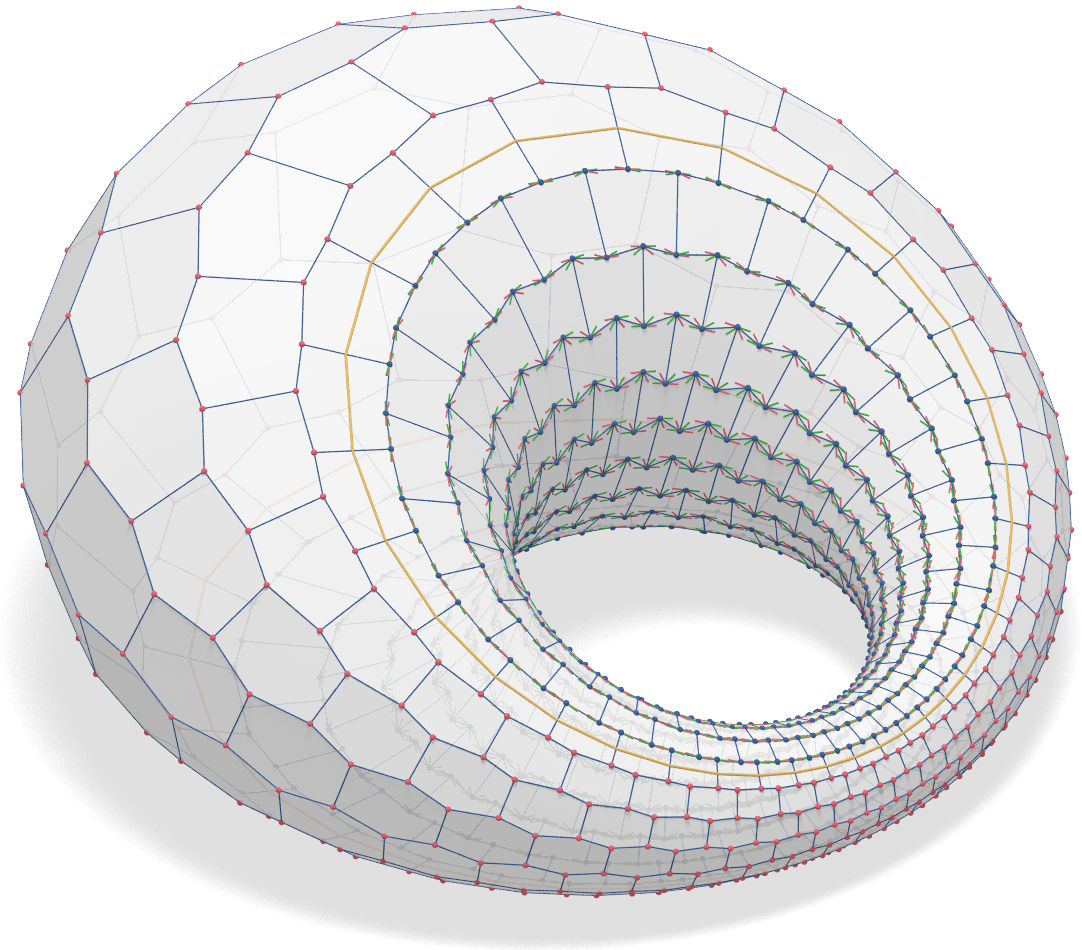A smooth Dupin cyclide composed of hexagons: The discrete parabolic curve is yellow, the discrete asymptotic directions are marked red and green.